# Speed Prior

## AI specialist Jürgen Schmidhuber on inductive inference, universal Solomonoff prior and measuring probability of different events

### videos | January 11, 2021

This lecture is part of the collaboration between Serious Science and the Technology Contests Up Great READ//ABLE.

Our next lecture is going to be about the speed prior which is really useful for inductive inference. What is inductive inference about? There is data coming in, and we can encode the incoming data in terms of bit string: all the videos, all the audio that is coming into a learning system can be encoded by some bit string x, and then at a given moment in time you have observed x and then you want to predict the future because machine learning is about predicting future things, unseen things from past experience. So the big question is now, given the x that I have seen so far, what is the future that I have to expect? And using Bayes’ rule what we can do is we can say: okay, let’s look at the possible futures y, so the entire life will be the combination of x and y, and now the question is, what is the most likely y that we can observe given the x that we already have seen? And then Bayes basically says, the probability of y coming after x, of x being followed by y, is proportional to the a priori probability of xy taken together.Philosopher Denis Bonnay on Kant's "Critique of Pure Reason", logical inferences, and attempts to divide mathematics and logic
Solomonoff at some point in the 60s came up with an ingenious way of optimally predicting futures in settings where the only assumption is that the x‘s and y‘s are drawn from a computable probability distribution. He had an uncomputable way of devising a universal prior which is known as the universal Solomonoff prior. This universal prior dominates on computer probabilites in the sense that it assigns as much probability to any x as any computable prior safe for a constant factor which does not depend on the size of x or anything like that.

Now, having said that, let me point out that Solomonoff’s optimal but non-computable method for inductive interference indeed assumes that observation sequences x are drawn from a recursive prior distribution which is often called μ(x). μ(x) is the original prior distribution which we don’t know, so we don’t know what is the rule that defines the new data coming in based on the old data that we have seen already. So instead of using the unknown μ(x) we predict using the celebrated universal innumerable prior or sometimes called Solomonoff-Levin (semi)measure M(x) which for all x exceeds any recursive μ(x) which is the true distribution which we don’t know, say, for this constant factor independent of x. By the way, Ray Solomonoff was a visiting professor here in our lab in the early 2000s. Unfortunately, he passed away a couple of years later.

The simplicity measure M(x) of Solomonoff’s universal prior naturally implements Occam’s razor. Occam’s razor says, simple solutions are preferred over complex solutions. This notion of simplicity is closely related to K(x), the Kolmogorov complexity or algorithmic information of x. In fact, there’s a close connection between M(x), Solomonoff’s universal prior, and K(x), the Kolmogorov complexity of x. My previous postdoc Marcus Hutter has explored lots of these connections. Predictions based on M are optimal in a certain incomputable sense.

However, M, this innumerable universal prior, assigns high probability to certain data x that are extremely hard to compute, so although these x‘s are really hard to compute, they get a lot of a priori probability assigned through this M(x).

This doesn’t really match our intuitive notion of simplicity because our intuitive notion of simplicity has something to do with the complexity associated with computing, something from some other thing. So I suggested a more plausible measure, universal measure derived from the fastest way of computing data, not necessarily from the shortest way of computing data, the most compressed way but the fastest. In absence of any contrary physical evidence I assume that all of the physical world around us is generated by a computational process without any probabilistic interventions: just by a computer program that computes all of the data that’s coming in and that all possibly infinite sequences of observations are therefore computable in the limit. This assumption is more radical and stronger than the one of Ray Solomonoff who just assumed that the distribution is computable, but in his case you can have infinitely long histories of universes which cannot be computed by a finite program.

What I did I replaced Solomonoff’s measure N by the novel speed prior S under which the cumulative a priori probability of all data whose computation through an optimal algorithm requires more than order of n resources, this asymptotic optimality, O notation, so more than O(n) resources is 1/n. That’s the speed prior.

To evaluate the plausibility of this, consider that most data generated on your own computer are computable within a few microseconds, and some of them take a few seconds, and a few data files take a couple of hours, and very few take days etc.

So in that sense the speed prior seems to be a quite reasonable way of translating resource limitations into a priori probabilities that should be used for inductive inference.

If you don’t predict the future through the Solomonoff’s universal measure, but instead use the speed prior, then you get different types of predictions, more precise predictions. Let me immediately go to an extreme application. There is no physical evidence against the assumption that the entire history of our Universe is computable through a finite program, and the most important goal of physics is to find that (hopefully short) program. We assume that this program is sampled according to the speed prior, according to S, or according to some less dominant prior which is dominated by the S prior which corresponds to some sub-optimal computation of the history.

The legendary Konrad Zuse was the first scientist who seriously suggested that the entire universe is being computed on a grid of computers, or a cellular automata, as it is now called. Compare the related work by Edward Fredkin who initiated the translation of Zuse’s 1969 book ‘Rechnender Raum‘, ‘Calculating Space’ where he presented this thesis.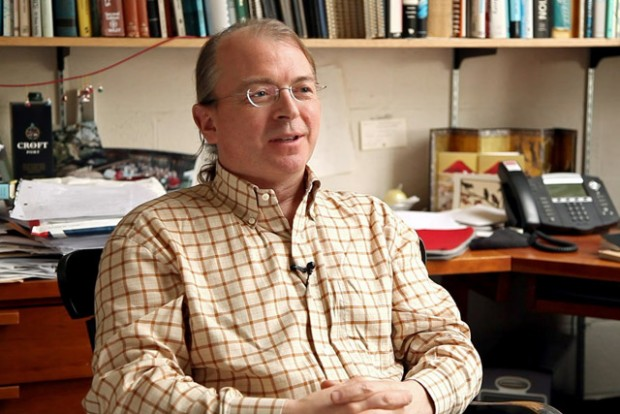Physicist Seth Lloyd on quantum mechanics, classical vs quantum computers and the “universal digital computation”
Under our assumptions of a computable universe, we can immediately predict, using the speed prior, that, first of all, that our universe, as it is, is not going to get many times older than it is now: that’s one interesting prediction. Another important prediction: large-scale quantum computation that everybody is hoping for is not going to work well. Maybe the adiabatic and certain types of quantum computation that are about simulating quantum systems may work nicely, but the really desirable universal quantum computation that would be used to break cryptographic codes and everything is not going to work well essentially because it would require too many computational resources in interfering parallel universes. The speed prior would assign low probability to that.

The third prediction is that any apparent randomness in any physical observation such as beta decay is in fact due not to true randomness, but to some yet unknown but fast pseudo-random generator which we should try to discover. Maybe the pseudo random generator is the short program that computes π, the digits of π: if you print them out, they look random, but they are not. Yes, every sequence of three numbers, like ‘596’ appears roughly once every one thousand times; however, it is not really random because there’s a very short program of π that computes all of that. The prediction is that everything that seems random at the moment is at some point going to turn out to be non-random and computed by a deterministic random number generator.

These ideas have recently attracted a lot of attention, and you can read more about that in the paper on the speed prior, but also in the even older paper I wrote in 2000 on the algorithmic theories of everything which then as a spin-off created the speed prior paper.

Did you like it? Share it with your friends!
Published items
0779
To be published soon
+92

#### Most viewed

• 1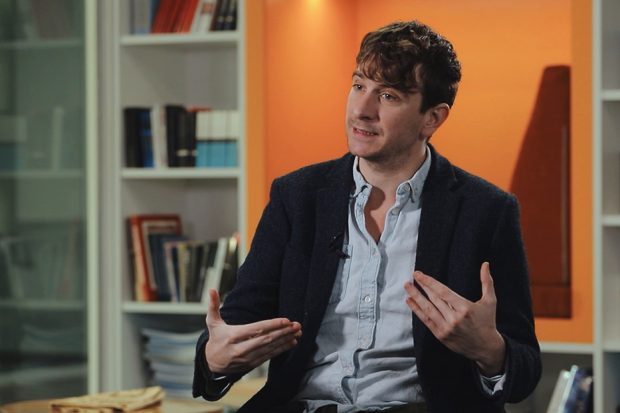Peter Jones
• 2John Worrall
• 3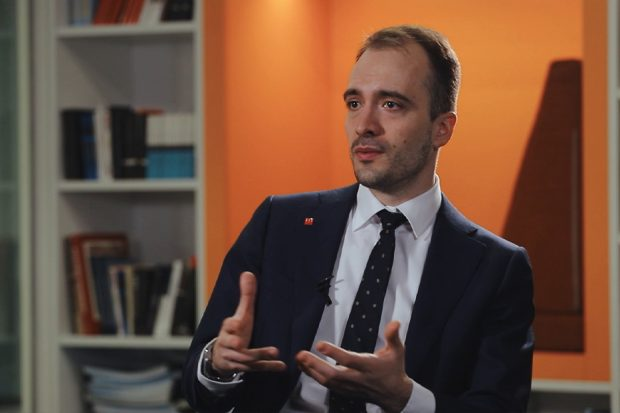Daniel Kontowski
• 4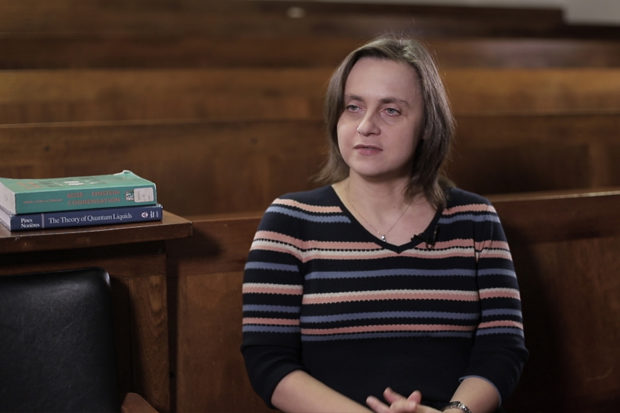Marzena Szymanska
• 5Jonathan Birch
• 6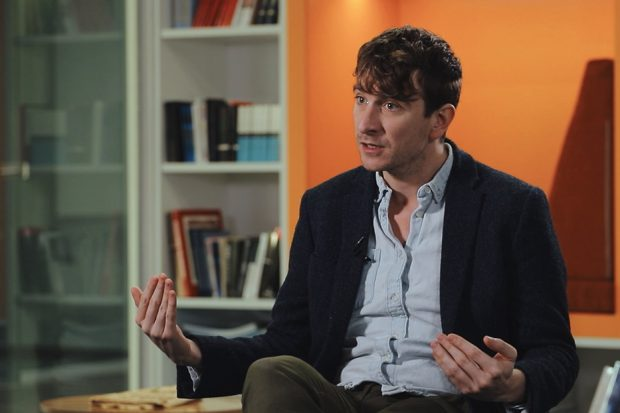Peter Jones
• 7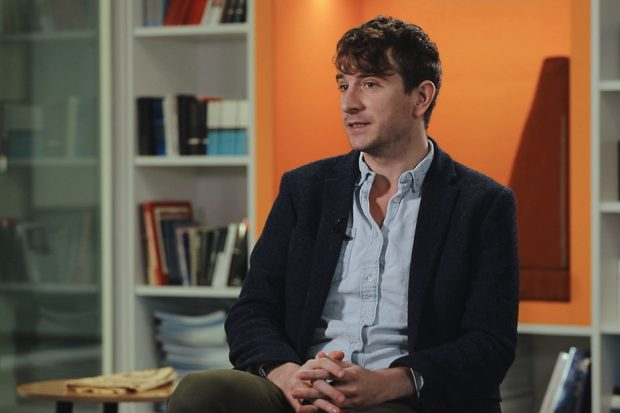Peter Jones
• 8Jeffrey Taylor
• 9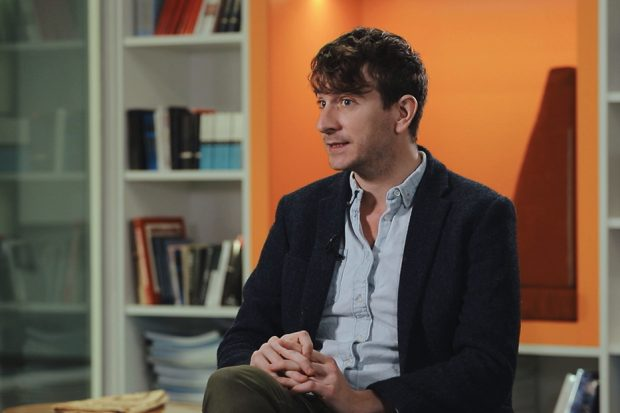Peter Jones
•